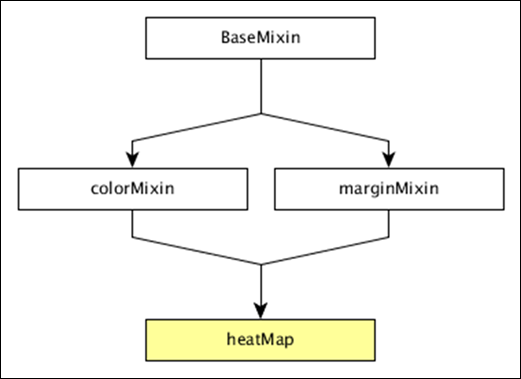# DC.js - Heat Map

A Heat Map is a graphical representation of data in the form of a map, in which data values are represented as colors. This chapter explains about a heat map in detail.

Before moving on to draw a heat map, we should understand the dc.heatMap class and its methods. The dc.heatMap uses mixins to get the basic functionality of drawing a chart, which are listed below −

• dc.colorMixin
• dc.marginMixin
• dc.baseMixin

The complete class diagram of the dc.heatMap is as follows −The dc.heatMap gets all the methods of the above-specified mixins. It has its own methods to draw the heat map, which are explained below −

### boxOnClick( [handler])

This method is used to get or set the handler, when an individual cell is clicked in the heatmap.

### cols( [cols])

This method is used get or set the keys to create the columns of the heatmap.

### colsLabel( [label])

This method is used to get or set the column label, which is represented as the column name. Similarly, we can perform a row label as well.

### rows( [rows])

This method is used to get or set the values used to create the rows of the heatmap.

### xAxisOnClick( [handler])

This method is used to get or set the handler, when a column tick is clicked in the x-axis.

This method is used to set the X border radius. If the value is set to 0, then you will get full rectangles.

## Draw a Heatmap

Let us draw a heatmap in DC. To do this, we need to follow the steps given below −

### Step 1: Define a variable

Let us define a variable as shown below −

```var chart = dc.heatMap('#heatmap');
```

Here, the heatMap function is mapped with the id heatmap.

### Step 2: Read the data

Read the data from the howell1.csv file as shown below −

```d3.csv("data/howell1.csv", function(errors, people) {
var mycrossfilter = crossfilter(people);
}
```

Here, we have used the same howell1.csv file and it looks as shown below −

```"height","weight","age","male"
151.765,47.8256065,63,1
139.7,36.4858065,63,0
136.525,31.864838,65,0
156.845,53.0419145,41,1
145.415,41.276872,51,0
163.83,62.992589,35,1
149.225,38.2434755,32,0
168.91,55.4799715,27,1
147.955,34.869885,19,0
165.1,54.487739,54,1
154.305,49.89512,47,0

......................
......................
```

### Step 3: Fetch the records

Let us fetch the records using the coding given below −

```people.forEach(function(x) {
x.age = Math.floor(x.age) + 1;
x.heightRange = Math.floor(x.height / 10) + 1;
x.weightRange = Math.floor(x.weight / 10) + 1;
if(x.male == 1) {
x.gender = 1;
} else {
x.gender = 2;
}
});
```

Here, we have checked the gender and have set the height and width range of the x-axis by using the above formula.

### Step 4: Set the dimension

You can set the dimension using the coding given below −

```var ageDimension = mycrossfilter.dimension(function(data) {
return [+data.gender, +data.heightRange];
});
```

After the dimension has been assigned, group the gender using the coding given below −

```var genderGroup = genderDimension.group().reduceCount();
```

### Step 5: Generate a chart

Now, generate a heatmap using the coding given below −

```chart
.width(20 * 45 + 80)
.height(2 * 45 + 40)
.dimension(ageDimension)
.group(ageGroup)
.keyAccessor(function(d) { return +d.key; })
.valueAccessor(function(d) { return +d.key; })
.colorAccessor(function(d) { return +d.value; })
.title(function(d) {
return "Height Range:   " + ((d.key - 1) * 10) + " - " + (d.key * 10) + "cm\n" +
"Gender:  " + (d.key == 1 ? "Male" : "Female") + "\n" +
"Count: " + (d.value) + " count";
})
.calculateColorDomain()

chart.render();
});
```

Here,

• We have assigned the chart width as 20 × 45 + 80 and height as 2 × 45 + 40.
• Then we have assigned the gender dimension and group.
• Key and value accessor returns the key and value from the heatmaps.
• We have to use the colorAccessor() function to return the color.
• Finally, set the title and render the chart.

### Step 6: Working example

The complete coding is as follows. Create a web page heatmap.html and add the following changes to it.

```<html>
<title>DC heat map Sample</title>
<link rel = "stylesheet" type = "text/css" href = "css/bootstrap.css">
<link rel = "stylesheet" type = "text/css" href = "css/dc.css"/>

<script src = "js/d3.js"></script>
<script src = "js/crossfilter.js"></script>
<script src = "js/dc.js"></script>

<body>
<div>
<div id = "heatmap"></div>
</div>

<script language = "javascript">
var chart = dc.heatMap('#heatmap');

d3.csv("data/howell1.csv", function(errors, people) {
var mycrossfilter = crossfilter(people);

people.forEach(function(x) {
x.age = Math.floor(x.age) + 1;
x.heightRange = Math.floor(x.height / 10) + 1;
x.weightRange = Math.floor(x.weight / 10) + 1;
if(x.male == 1) {
x.gender = 1;
} else {
x.gender = 2;
}
});

var ageDimension = mycrossfilter.dimension(function(data) {
return [+data.gender, +data.heightRange];
});

var ageGroup = ageDimension.group().reduceCount();
chart
.width(20 * 45 + 80)
.height(2 * 45 + 40)
.dimension(ageDimension)
.group(ageGroup)
.keyAccessor(function(d) { return +d.key; })
.valueAccessor(function(d) { return +d.key; })
.colorAccessor(function(d) { return +d.value; })
.title(function(d) {
return "Height Range:   " + ((d.key - 1) * 10) + " - " +
(d.key * 10) + "cm\n" +
"Gender:  " + (d.key == 1 ? "Male" : "Female") + "\n" +
"Count: " + (d.value) + " count";})
.calculateColorDomain()

chart.render();
});
</script>
</body>
</html>
```

Now, request the browser and we will see the following response.